﻿ 一种邻接区域平面元融合的桥面分割方法文章快速检索 高级检索
 大地测量与地球动力学2022, Vol. 42Issue (8): 863-869  DOI: 10.14075/j.jgg.2022.08.017### 引用本文ZHANG Xingyan, LI Qi, LIANG Dong, et al. A Bridge Deck Segmentation Method Based on Fusion of Plane Elements in Adjacent Regions[J]. Journal of Geodesy and Geodynamics, 2022, 42(8): 863-869.### Foundation support

National Natural Science Foundation of China, No. 51978236; Science and Technology Development Program of Tianjin Municipal Transportation Commission, No. 2019-06.

### Corresponding author

LI Qi, PhD, professor, majors in radar signal processing, E-mail: liqi@hebut.edu.cn.

### 第一作者简介

ZHANG Xingyan, postgraduate, majors in point cloud data processing and application, E-mail: hebut_zxy@163.com.

### 文章历史

1. 河北工业大学电子信息工程学院，天津市西平道5340号，300401;
2. 河北工业大学土木与交通学院，天津市西平道5340号，300401

1 桥面分割方法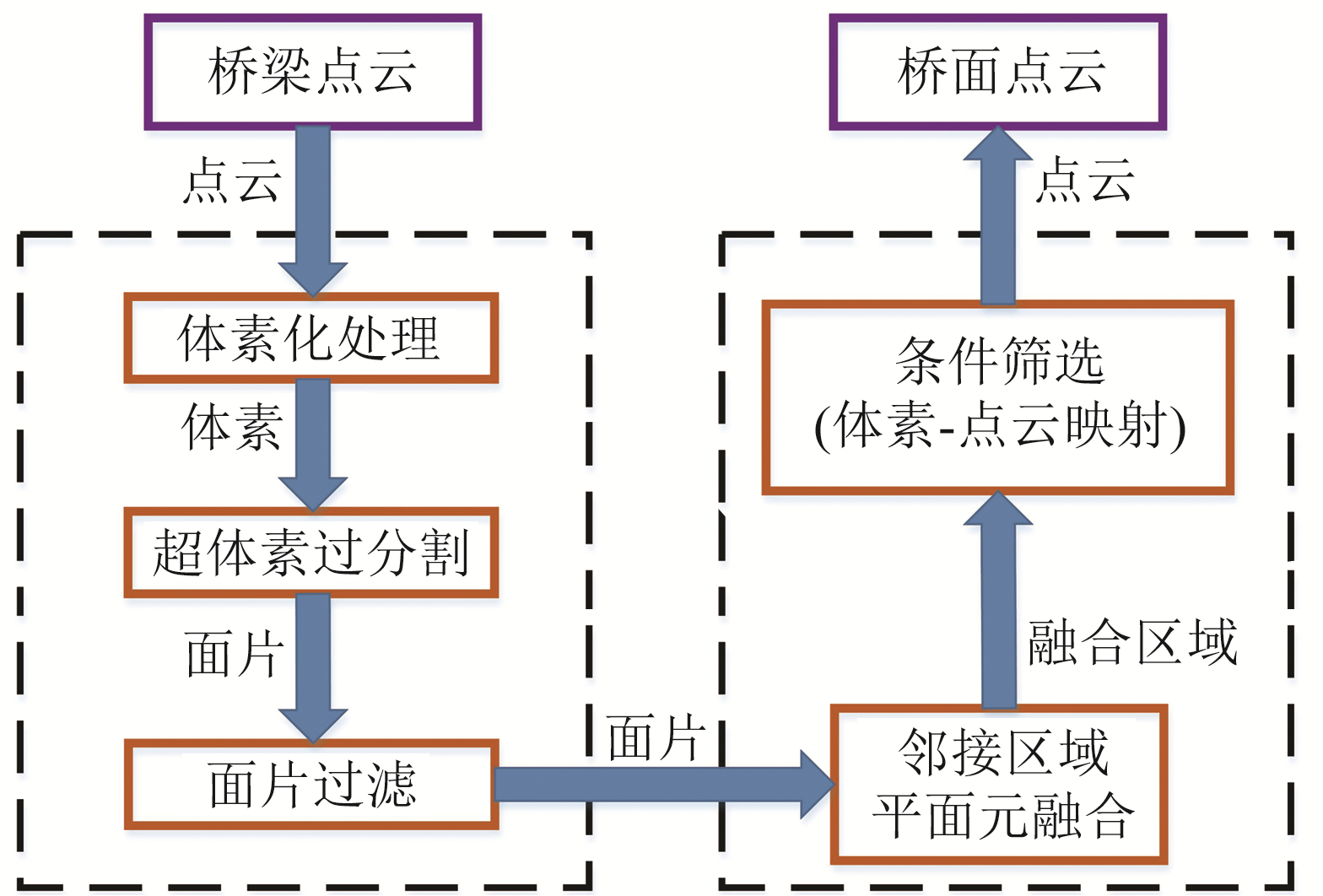图 1 桥面分割方法流程 Fig. 1 Flow chart of bridge deck segmentation method
1.1 超体素过分割

1) 以种子分辨率Rseed为单位建立若干初始种子。

2) 以Rsearch为搜索半径，计算种子范围内体素的数量，过滤掉小于阈值的种子，去除孤立点。

3) 以种子为中心逐层向外搜索，使用局部K均值聚类迭代生长法更新聚类中心，计算邻接体素与聚类中心所有体素之间相似性距离的平均值，将平均距离最近的邻接体素加入聚类。

4) 重复步骤3)直到无其他邻接体素或达到最大搜索范围，结束增长，获得超体素面片。面片中心点由靠近超体素质点的体素表示。

 $D=\sqrt{w_{s} \frac{D_{s}^{2}}{3 R_{\text {seed }}^{2}}+w_{n} D_{n}^{2}}$ (1)
 $D_{s}=\sqrt{\left(x_{i}-x_{j}\right)^{2}+\left(y_{i}-y_{j}\right)^{2}+\left(z_{i}-z_{j}\right)^{2}}$ (2)
 $D_{n}=1-\cos \left(\boldsymbol{n}_{i}, \boldsymbol{n}_{j}\right)$ (3)

1.2 面片过滤

 $\boldsymbol{n}_{i}^{*}=\left\{\begin{array}{l} \boldsymbol{n}_{i}, \boldsymbol{n}_{i} \cdot \boldsymbol{e}_{y} \geqslant 0 \\ -\boldsymbol{n}_{i}, \boldsymbol{n}_{i} \cdot \boldsymbol{e}_{y}<0 \end{array} i=1, 2, 3, \cdots, M\right.$ (4)

 $\left\{\begin{array}{l} \boldsymbol{n}_{\text {面片 }}^{*}=w_{0} \boldsymbol{n}_{0}^{*}+\sum\limits_{j=1}^{L} w_{j} \frac{1}{N_{j}} \sum\limits_{i=1}^{N_{j}} \boldsymbol{n}_{(j, i)}^{*} \\ w_{0}+\sum\limits_{j=1}^{L} w_{j}=1 \end{array}\right.$ (5)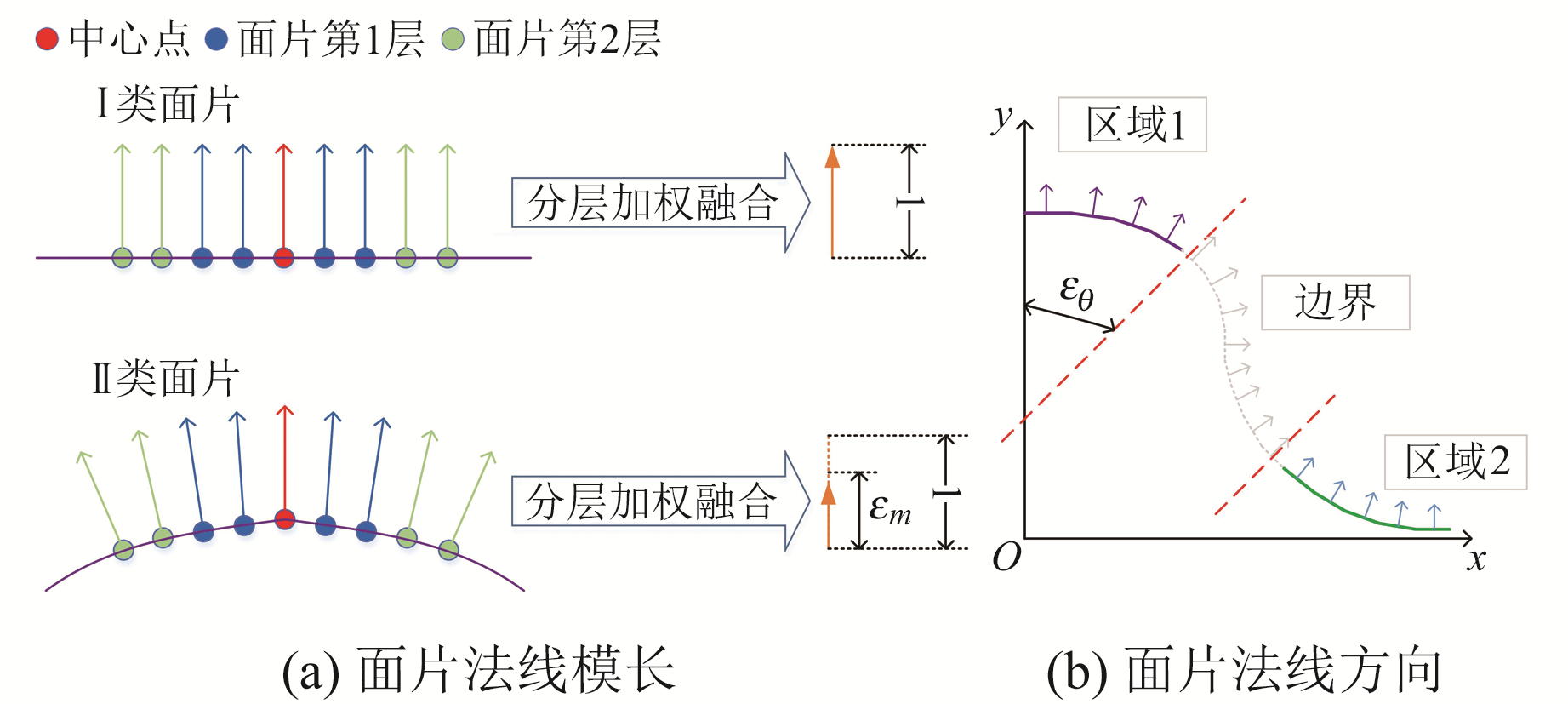图 2 面片过滤的2种指标 Fig. 2 Two indicators of patch filter

 $\left\{\begin{array}{l} \left\|\boldsymbol{n}_{\text {面片 }}^{*}\right\|>\varepsilon_{m} \\ R\left(\boldsymbol{n}_{\text {面片 }}^{*}, \boldsymbol{e}_{y}\right)<\varepsilon_{\theta} \end{array}\right.$ (6)
1.3 邻接区域平面元融合

1) 为提高面片的增长效率，选取所有曲率最小的面片作为初始种子。

2) 从区域A中取出初始种子，将初始种子加入到一组区域Rc中，并加入种子队列Sc

3) 开始区域增长：

① 从种子队列Sc中取出队头的种子面片Sci

② 根据面片邻接关系索引表获得种子面片Sci的所有邻接面片，存于邻接区域Bc中；

③ 计算种子面片Sci与其邻接面片Bcj的相似性度量Sij，若Sij大于相似性度量阈值Sth，则加入该组区域Rc中；若面片曲率γ大于曲率阈值γth，则将该邻接面片Bcj加入种子队列Sc的队尾；

④ 清空邻接区域Bc，返回步骤①，直到种子队列Sc为空。

4) 将增长后的区域Rc添加至区域B中，清空区域Rc，返回步骤1)，直到区域A为空。至此，平面元融合结束，获得多组融合区域B

 $S_{n}=|\cos \alpha|=\left|\frac{\boldsymbol{n}_{i} \cdot \boldsymbol{n}_{j}}{\left|\boldsymbol{n}_{i}\right|\left|\boldsymbol{n}_{j}\right|}\right|$ (7)

 $k= \begin{cases}\text { true } & R\left(\overrightarrow{M_{0} M}, \overrightarrow{P_{0} P}\right)>\frac{\pi}{2} \\ \text { false } & R\left(\overrightarrow{M_{0} M}, \overrightarrow{P_{0} P}\right) \leqslant \frac{\pi}{2}\end{cases}$ (8)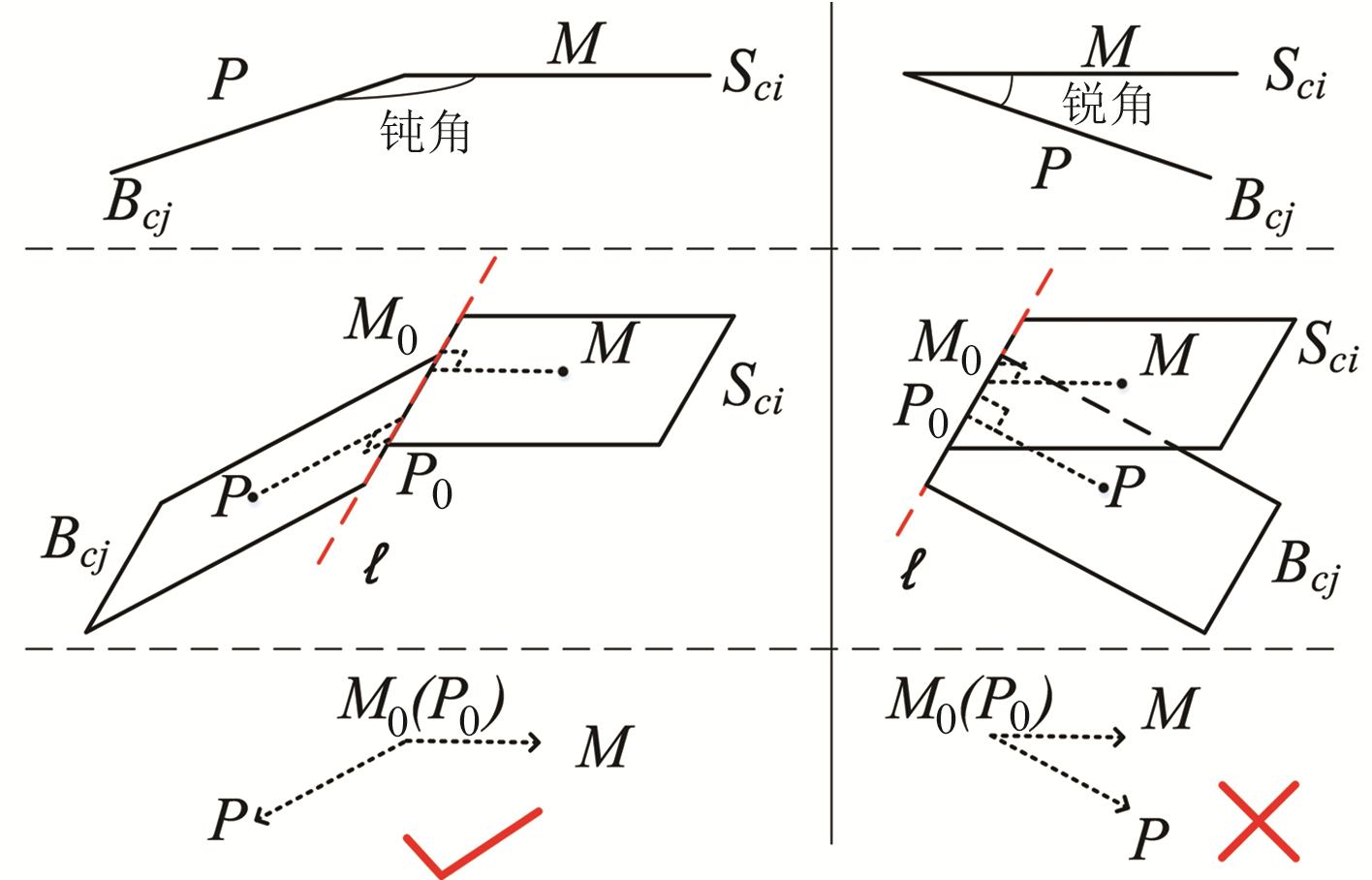图 3 相邻面片的夹角 Fig. 3 The angle between adjacent patch

 $S_{i j}=\left\{\begin{array}{l} S_{n}, \alpha \leqslant \alpha_{\mathrm{th}} \\ k \cdot S_{n}, \alpha>\alpha_{\mathrm{th}} \end{array}\right.$ (9)

1.4 桥面点云

1) 桥梁点云y轴垂直于地面，y坐标最小值为Ymin，统计融合区域点云的y坐标，计算平均值Ymean_n

2) 融合区域的点云向地面xoz投影，计算x轴方向的最大值Xmax_n和最小值Xmin_n，计算z轴方向的最大值Zmax_n和最小值Zmin_n

3) 对投影区域进行正方形网格划分，设置每个网格边长为Gsize，统计每个网格中投影点的数量，生成点云分布矩阵。

4) 将点云分布矩阵转化为灰度图像，为更直观地展示投影形状，使用大津法设置阈值，进行二值化处理，即图像中大于阈值的像素设为1，最后生成二值图。

5) 统计二值图中值为1的像素数，记为投影面积Sp_n。使用旋转主轴法计算二值图的最小外接矩形，统计最小外接矩形所占用的像素数，记为外接矩形面积Srect_n

6) 3个指标的计算公式为：

 $D_{\text {矩 }\_n}=\frac{S_{p_{-} n}}{S_{\text {rect_ } n}}$ (10)
 $H_{\text {mean_ } n}=\frac{Y_{\text {mean }\_n}-Y_{\text {min }}}{Y_{\text {mean_max }}-Y_{\text {min }}}$ (11)
 $R_{n}^{\prime}=1.0-0.02\left(R_{n}-1\right)$ (12)

 $P_{n}=D_{\text {矩_ } n} \cdot H_{\text {mean_ } n} \cdot R_{n}^{\prime}$ (13)
2 实验与分析 2.1 拱形桥面分割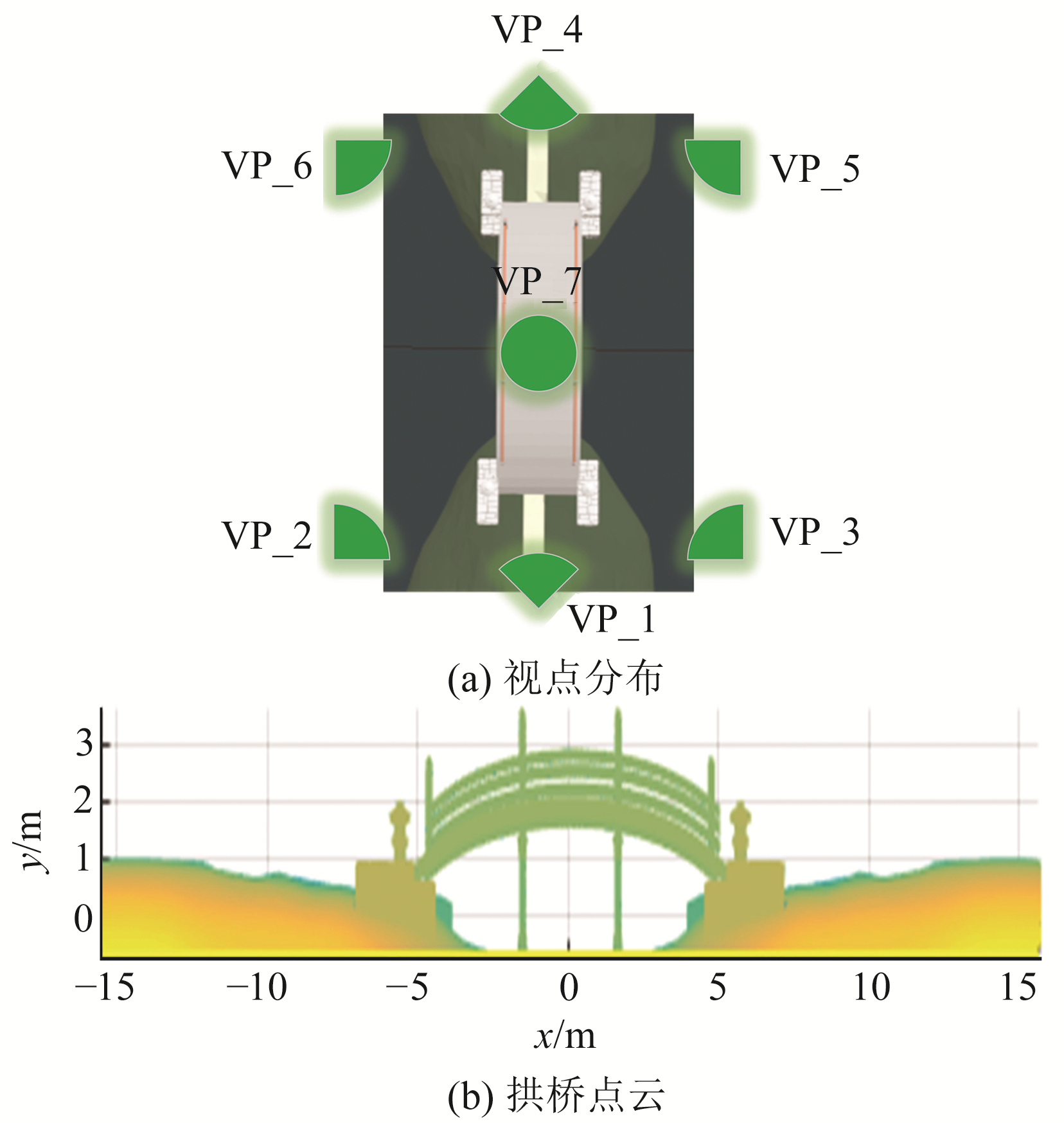图 4 拱形桥实验数据 Fig. 4 Experimental data of arched bridge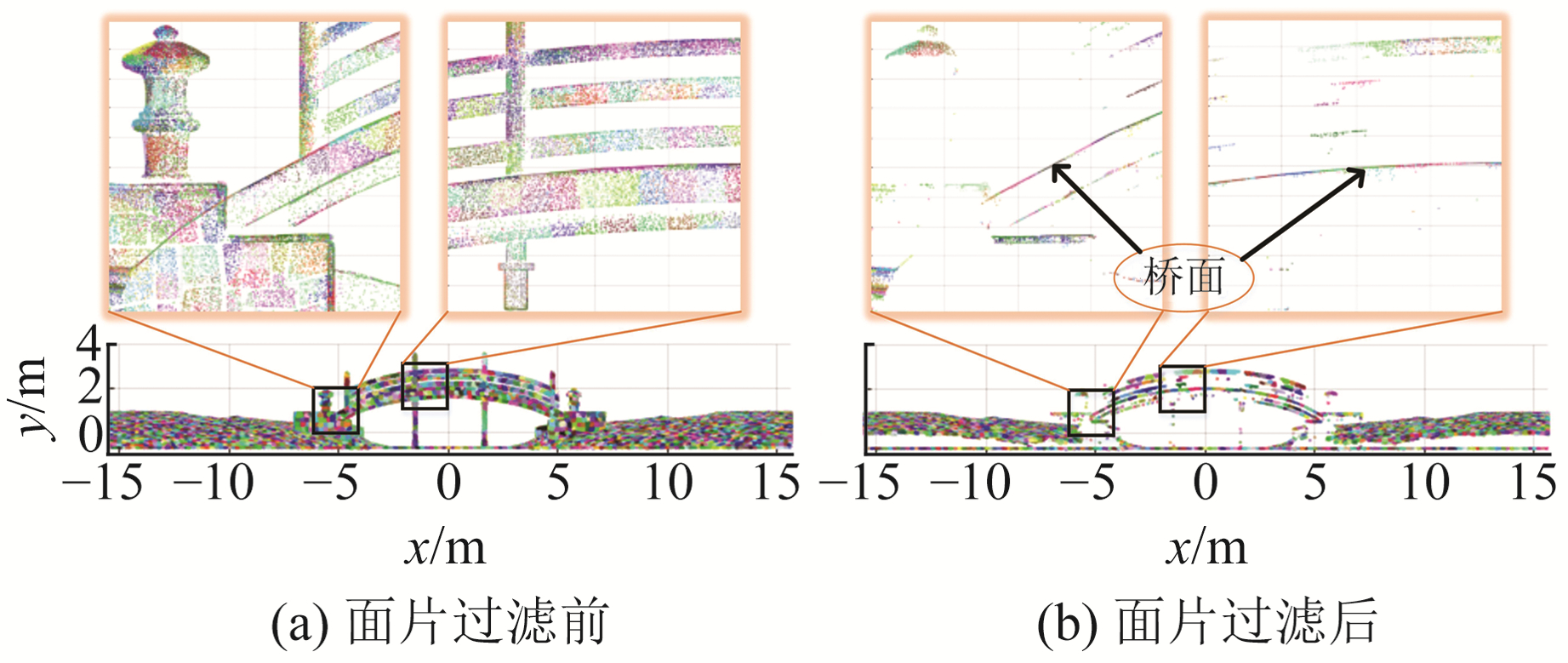图 5 面片过滤 Fig. 5 Patch filtering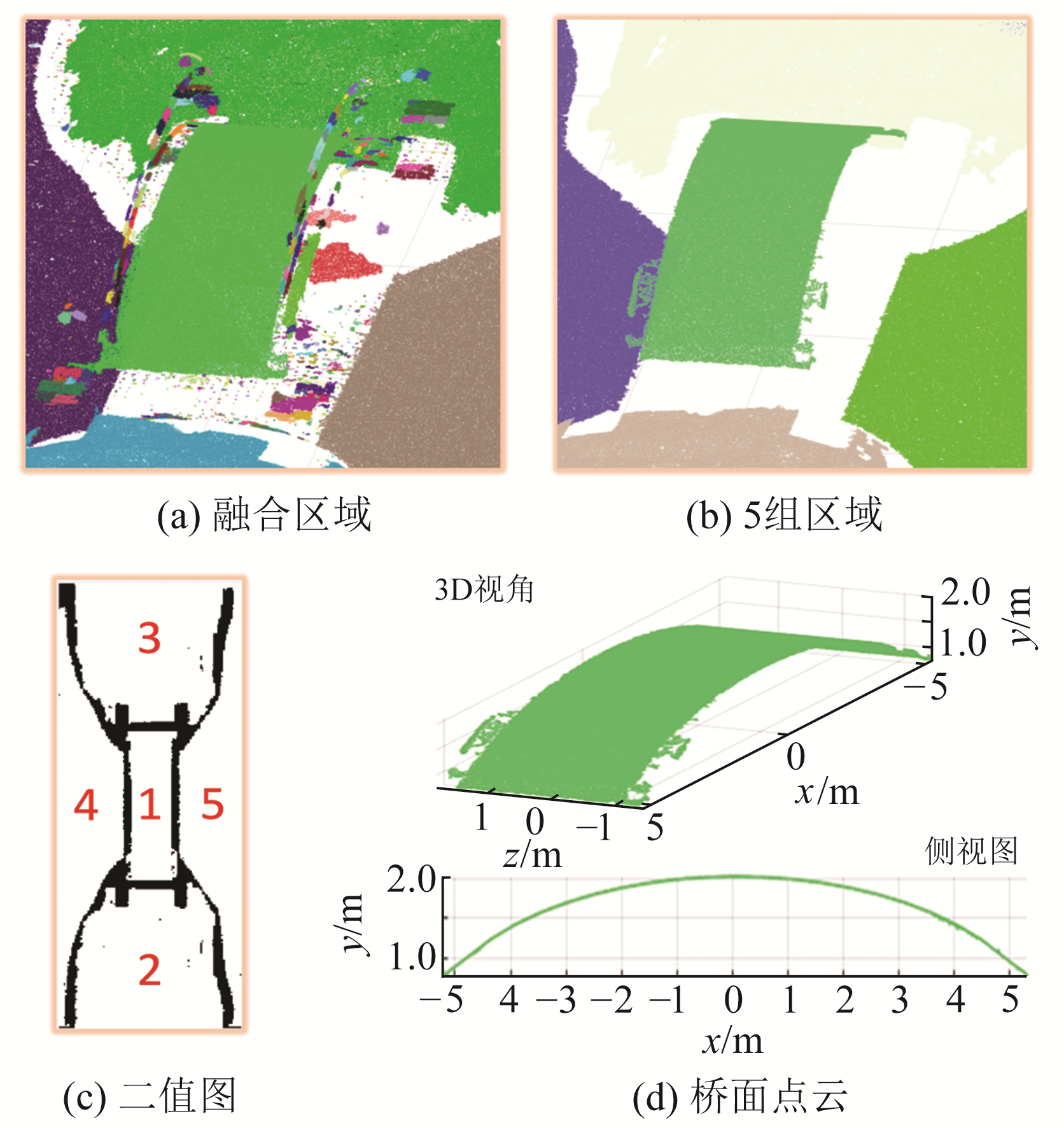图 6 筛选过程 Fig. 6 Screening process表 1 5组区域的评价指标 Tab. 1 Evaluation indicators for five groups of regions
2.2 其他桥梁桥面分割图 7 钢筋桥和斜拉桥的分割实验 Fig. 7 Segmentation experiments of reinforced and cable-stayed bridges表 2 调试参数 Tab. 2 Debug parameter表 3 桥面点云的数量 Tab. 3 Number of point clouds on the bridge

2.3 与其他方法的比较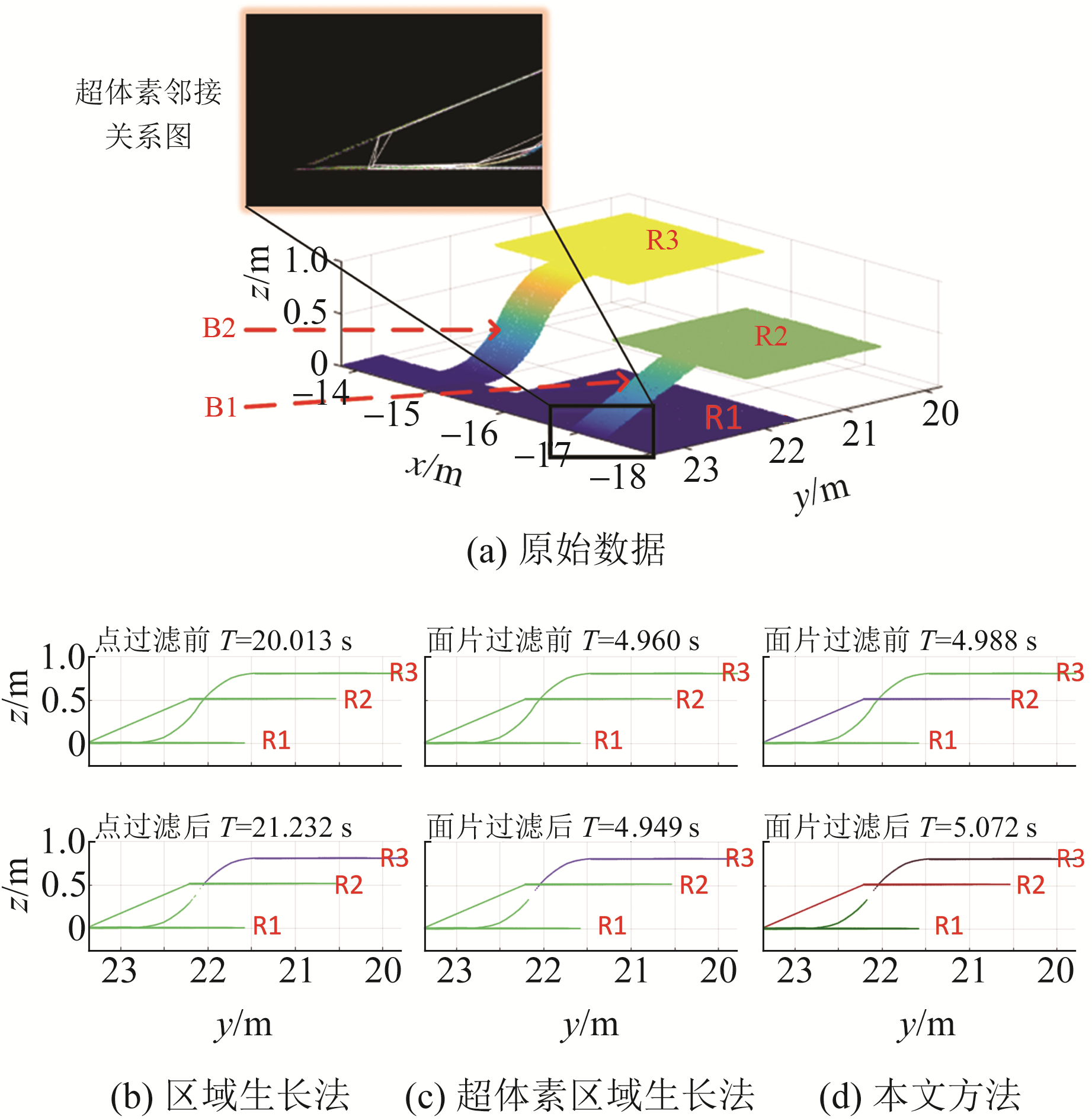图 8 对比实验 Fig. 8 Comparison experiment

3 结语

  徐进军, 郭鑫伟, 廖骅, 等. 基于地面三维激光扫描的桥梁挠度变形测量[J]. 大地测量与地球动力学, 2017, 37(6): 609-613 (Xu Jinjun, Guo Xinwei, Liao Hua, et al. The Test on Bridge Deflection Deformation Monitoring by Terrestrial Laser Scanning[J]. Journal of Geodesy and Geodynamics, 2017, 37(6): 609-613) (0)  赖亦斌, 陆声链, 钱婷婷, 等. 植物三维点云分割[J]. 应用科学学报, 2021, 39(4): 660-671 (Lai Yibin, Lu Shenglian, Qian Tingting, et al. Three-Dimensional Point Cloud Segmentation for Plants[J]. Journal of Applied Sciences, 2021, 39(4): 660-671 DOI:10.3969/j.issn.0255-8297.2021.04.013) (0)  张凯, 于春磊, 赵亚丽, 等. 基于自适应阈值的三维激光点云地面分割算法研究[J]. 汽车工程, 2021, 43(7): 1 005-1 012 (Zhang Kai, Yu Chunlei, Zhao Yali, et al. Research on Ground Segmentation Algorithm Based on Adaptive Thresholds for 3D Laser Point Clouds[J]. Automotive Engineering, 2021, 43(7): 1 005-1 012) (0)  周钦坤, 岳建平, 李乐乐, 等. 一种分步式建筑物屋顶面点云高精度提取方法[J]. 大地测量与地球动力学, 2021, 41(6): 633-638 (Zhou Qinkun, Yue Jianping, Li Lele, et al. A Stepwise High-Precision Extraction for Point Cloud of Building Roof[J]. Journal of Geodesy and Geodynamics, 2021, 41(6): 633-638) (0)  Hamid L F. Structural-Based Point Cloud Segmentation of Highly Ornate Building Facades for Computational Modelling[J]. Automation in Construction, 2019, 108 (0)  熊汉江, 郑先伟, 丁友丽, 等. 基于2D-3D语义传递的室内三维点云模型语义分割[J]. 武汉大学学报: 信息科学版, 2018, 43(12): 2 303-2 309 (Xiong Hanjiang, Zheng Xianwei, Ding Youli, et al. Semantic Segmentation of Indoor 3D Point Cloud Model Based on 2D-3D Semantic Transfer[J]. Geomatics and Information Science of Wuhan University, 2018, 43(12): 2 303-2 309) (0)  Poz A P D, Ywata M S Y. Adaptive Random Sample Consensus Approach for Segmentation of Building Roof in Airborne Laser Scanning Point Cloud[J]. International Journal of Remote Sensing, 2020, 41(6): 2 047-2 061 DOI:10.1080/01431161.2019.1683644 (0)  Zhao X K, Li H, Zhu Q B, et al. Automatic Sweet Pepper Detection Based on Point Cloud Images Using Subtractive Clustering[J]. International Journal of Agricultural and Biological Engineering, 2020, 13(3): 154-160 DOI:10.25165/j.ijabe.20201303.5460 (0)  杨琳, 翟瑞芳, 阳旭, 等. 结合超体素和区域增长的植物器官点云分割[J]. 计算机工程与应用, 2019, 55(16): 197-203 (Yang Lin, Zhai Ruifang, Yang Xu, et al. Segmentation of Plant Organs Point Clouds through Super Voxel-Based Region Growing Methodology[J]. Computer Engineering and Applications, 2019, 55(16): 197-203 DOI:10.3778/j.issn.1002-8331.1805-0221) (0)  Boulch A, Guerry J, Saux B, et al. SnapNet: 3D Point Cloud Semantic Labeling with 2D Deep Segmentation Networks[J]. Computers and Graphics, 2018, 71: 189-198 DOI:10.1016/j.cag.2017.11.010 (0)  Xu Y S, Yao W, Hoegner L, et al. Segmentation of Building Roofs from Airborne LiDAR Point Clouds Using Robust Voxel-Based Region Growing[J]. Remote Sensing Letters, 2017, 8(11): 1 062-1 071 DOI:10.1080/2150704X.2017.1349961 (0)  董震, 杨必胜. 车载激光扫描数据中多类目标的层次化提取方法[J]. 测绘学报, 2015, 44(9): 980-987 (Dong Zhen, Yang Bisheng. Hierarchical Extraction of Multiple Objects from Mobile Laser Scanning Data[J]. Acta Geodaetica et Cartographica Sinica, 2015, 44(9): 980-987) (0)  卜文斌, 游福成, 李泉, 等. 一种基于大津法改进的图像分割方法[J]. 北京印刷学院学报, 2015, 23(4): 76-78 (Bu Wenbin, You Fucheng, Li Quan, et al. An Improved Image Segmentation Method Based on Otsu[J]. Journal of Beijing Institute of Graphic Communication, 2015, 23(4): 76-78 DOI:10.3969/j.issn.1004-8626.2015.04.018) (0)  张法全, 王国富, 曾庆宁, 等. 利用重心原理的图像目标最小外接矩形快速算法[J]. 红外与激光工程, 2013, 42(5): 1 382-1 387 (Zhang Faquan, Wang Guofu, Zeng Qingning, et al. New Algorithm for Minimum Enclosing Rectangle of the Object in the Image Region Based on Center-of-Gravity Principle[J]. Infrared and Laser Engineering, 2013, 42(5): 1 382-1 387) (0)
A Bridge Deck Segmentation Method Based on Fusion of Plane Elements in Adjacent Regions
ZHANG Xingyan1LI Qi1LIANG Dong2     PU Jie2
1. School of Electronic and Information Engineering, Hebei University of Technology, 5340 Xiping Road, Tianjin 300401, China;
2. School of Civil and Transportation Engineering, Hebei University of Technology, 5340 Xiping Road, Tianjin 300401, China
Abstract: Aiming at the problem of bridge deck segmentation of bridge point cloud, we propose a bridge deck segmentation method based on the fusion of plane elements in adjacent regions. Firstly, the point cloud is voxelized to obtain the voxel point cloud, and the supervoxel space and normal constraint criteria are used to over-segment the bridge point cloud. Then, we use the direction of the patch normal and the model length to perform the patch filter to obtain the candidate patch containing the bridge deck. Finally, we perform the plane element fusion of the adjacent area on the candidate patch and use the statistical distribution method to filter the fusion area to obtain the bridge deck point cloud. Experiments show that the method in this paper can effectively segment the bridge deck of the bridge point cloud, has high stability, and retains the original data before voxelization. Compared with the region growing and the supervoxel region growing methods, the method in this paper integrates across regions. It has a better inhibitory effect and is more suitable for bridge deck segmentation of bridge point clouds.
Key words: bridge point cloud; supervoxel over-segmentation; patch filtering; plane element fusion; bridge deck segmentation